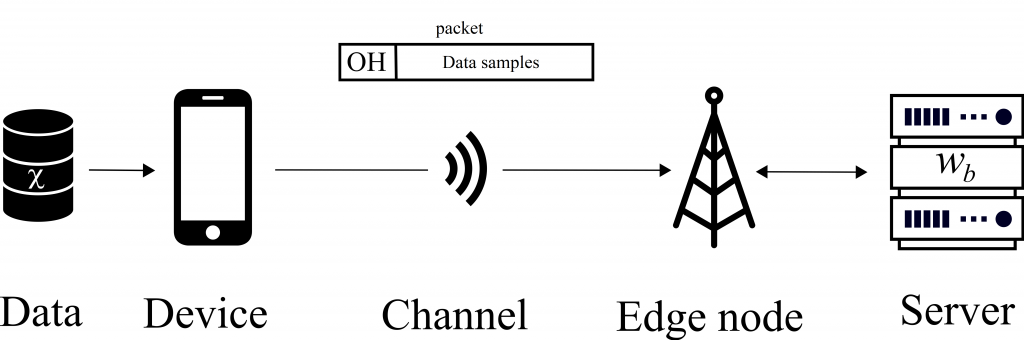ProblemFigure 1. Delay-constrained edge learning based on data received from a device.

The increasing number of connected devices has led to an explosion in the amounts of data being collected: smartphones, wearable devices and sensors generate data to an extent previously unseen. However, these devices often present power and computational capability constraints that do not allow them to make use of the data – for instance, to train Machine Learning (ML) models. In such circumstances, thanks to mobile edge computing, devices can rely on remote servers to perform the data processing (see Fig. 1). When the amount of data is large, or the access link slow, the amount of time required to transmit the data may be prohibitive. Given a delay constraint on the overall time available for both communication and learning, what is the joint communication-computation strategy that obtains the best performing ML model?

Pipelining communication and computationFigure 2. Transmission and training protocol.

In a recent work to be published in IEEE Communication Letters, we propose to pipeline communication and computation with an optimized block size. We consider an Empirical Risk Minimization (ERM) problem, for which learning is carried at the server side using Stochastic Gradient Descent (SGD). As the first data block arrives at the server, training of the ML model can start. This continues by fetching data from all the data blocks received thus far. To provide some intuition on the problem of optimizing the block size, communicating the entire data set first reduces the bias of the training process but it may not leave sufficient time for learning. Conversely, transmitting very few samples in each block will bias the model towards the samples sent in the first blocks, as many computation rounds will happen based on these samples.
We determine an upper bound on the expected optimality gap at the end of the time limit, which gives us an indication on how far we are from an optimal model. We can then minimize this bound with regard to the communication block size to obtain an optimized value.

Some resultsFigure 3. Training loss versus training time for different values of the block size. Solid line: experimental and theoretical optima.

Numerical experiments allowed us to compare the optimal block size found using the bound with a numerically determined optimal value found by running Monte Carlo experiments over all possible block sizes. Determining the optimal value through an extensive search over the possible block sizes allowed a gain of 3.8% in terms of the final training loss in one of our experiments (see Fig. 3). This small gain comes at the cost of a burdensome parameter optimization that took days on an HPC cluster. Minimizing the proposed bound takes seconds.
We further experimentally determined that our results, which were derived for convex loss functions satisfying the Polyak-Lojasiewicz condition, can be extended to non-convex models. As an example (not found in the paper), we studied the problem of training a multilayer perceptron with non-linear activations according to our scheme (see Fig. 4). Using the same dataset as described in the paper, we train a 2-layers perceptron with ReLU activation for the first layer and linear activation for the second. The experiments show a similar behaviour to the convex example discussed in the main text. In particular, the derived bound predicts well the existence of an optimum value of the block size (see crosses).Figure 4. Training loss versus block size for different overhead sizes, for an MLP with non-linear activations.

The full paper can be found here.